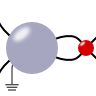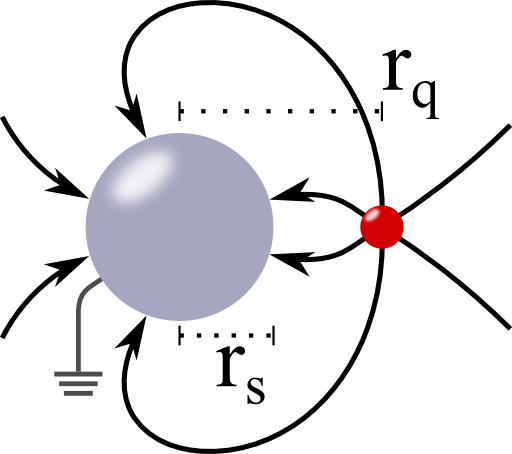A Metallic Sphere subject to a Point ChargeIn this problem we will encounter the main physical features of dielectric spheres - their induced field and polarizability. The result is of great interest: understanding the interaction of light with small particles is one of the main concerns of nanophotonics. It leads to astonishing applications like cloaking, improvement of solar cells and lithography with extreme resolution.

Problem StatementIn the origin of the coordinate system is a metallic sphere with radius $$r_{s}$$. At $$\mathbf{r}_{q}$$, there is a charge $$q$$. Calculate the resulting electrostatic potential for the cases if the sphere is grounded and if is isolated and charged with $$Q_{s}$$. Calculate the surface charge density $$\sigma$$ on the grounded sphere. You may want to proceed as follows:

1. Calculate the potential for the grounded sphere by the method of image charges and determine $$\sigma$$.
2. How does your obtained potential has to be modified if the sphere is not grounded but at some fixed potential $$V$$?
3. Relate the case of a fixed potential to that of an isolated charged sphere.

Background: "Ancient" and Modern Applications

A nice example for the field of a point charge in front of a charged metallic shell can be seen for example in the Van de Graaff generator. Everyone of us has seen these fancy images if someone gets charged by such a device and her/his hair follow the resulting field lines.

However, there are a lot of examples in modern science that make use of the results we are about to find. One of the main reasons is that the interaction of a charged body like an ion to a metallic sphere strongly depends on the distance between the two. We will see this both with respect to the magnitude and position of the image charge. This makes metallic spheres, or, generally, curved metallic nanoparticles a major candidate for sensor applications with a high spatial resolution. For more information I recommend the nice review article Plasmonic Materials, Advanced Materials 19, 2007 by Murray and Barnes.